# simple-3dviz

simple-3dviz is a lightweight and easy-to-use library that provides an easy interface for visualizing 3D objects with hundreds of thousands of vertices efficiently without the overhead of writing hundreds of lines of code. It can be used for visualizing various renderables such as meshes, point clouds, voxel grids, a set of geometric primitives etc.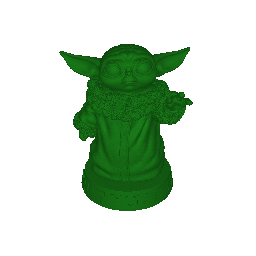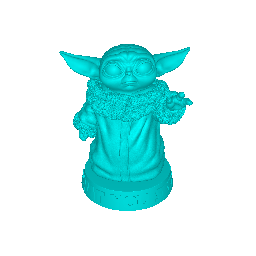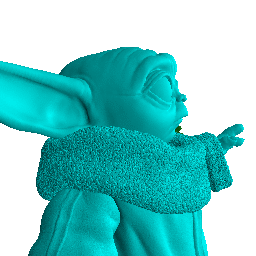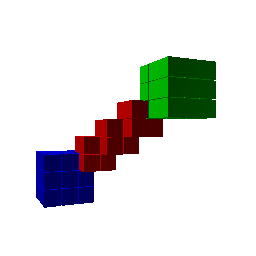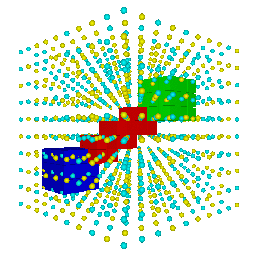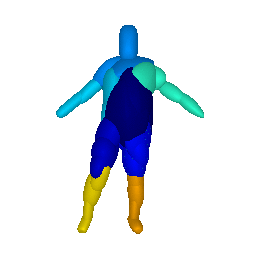## Installation Guide

simple-3dviz has the following dependencies:

The easiest way to install it is from PyPI with:

``````pip install --user simple-3dviz # --user is obviously optional
``````

In addition to the main library, we also provide two useful console applications that can be used for visualizing meshes (mesh_viewer) and 2D functions (func_viewer).

## Quick Start

Below, we show the basic functionalities of simple-3dviz, which include, but are not limited to:

``````from simple_3dviz import Mesh
from simple_3dviz.window import show
from simple_3dviz.utils import render

# We can load meshes from a file by specifying its path or by explicitely
# giving the vertices and the normals of the mesh you want to render
m = Mesh.from_file("models/baby_yoda.stl")

# Preview the mesh in an OpenGL window if you installed wxpython with pip
# Note that you can specify the size (size) and the background color
# (background) of the rendered window as well as the position of the camera in
# the scene (camera_position), its viewing direction (camera_target) and the 3d
# direction that indicates which direction is "up" (up_vector). Finally you can
# also specify the location of the light source as well a set of behaviours to
# be performed.
show(m, camera_position=(-60., -160, 120), camera_target=(0., 0, 40),
light=(-60, -160, 120))

# Our rendered mesh looks nice already but it is still not very accurate. This
# can be fixed by properly adjuasting the color of the input mesh through the
# color argument
m = Mesh.from_file("models/baby_yoda.stl", color=(0.1,0.5,0.1))

# We can specify various interesting behaviours while rendering our mesh
# Lets start by moving the camera around the object in a circular trajectory
from simple_3dviz.behaviours.movements import CameraTrajectory
from simple_3dviz.behaviours.trajectory import Circle

# The clockwise circular trajectory in 3D is defined by a 3D point that
# indicates the center of the circlular trajectory (center), a 3D point (point)
# and a 3D point that indicates the normal vector (normal). The 3D point point
# indicates the starting point of the trajectory
c = Circle(center=(0, 0, 120.), point=(-60, -160, 120.), normal=(0, 0, 1.))
ctrj = CameraTrajectory(c, speed=0.005)
show(m, camera_position=(-60., -160, 120), camera_target=(0., 0, 40),
light=(-60, -160, 120), behaviours=[ctrj])

# Nice, but unfortunately the light remains at a fixed position, which means
# that when the camera looks at the back of the object it is not illuminated.
# To fix this we can add another behaviour called LightToCamera.
from simple_3dviz.behaviours.misc import LightToCamera
show(m, camera_position=(-60., -160, 120), camera_target=(0., 0, 40),
light=(-60, -160, 120), behaviours=[ctrj, LightToCamera()])

# Note that we can also render the scene, without the need for any GUI
# environment using the render(...) function instead of the show(...) function.
# The render function takes the same arguments as the show function with an
# additional argument that indicates the number of frames to be rendered
# (n_frames).
# Saving the rendering results to the hard disk is implemented
# with a behaviour. This allows us to choose how to save the rendered frames
# and reuse the saving code for the show(...) pipeline.
from simple_3dviz.behaviours.io import SaveFrames
# To store the rendered frames to files, we can use the SaveFrames behaviour.
# We simply need to specify the path to save the rendered frames (path).
render(m,
behaviours=[
ctrj,
LightToCamera(),
SaveFrames("/tmp/frame_{:03d}.png", every_n=5)
],
n_frames=512,
camera_position=(-60., -160, 120), camera_target=(0., 0, 40),
light=(-60, -160, 120)
)

# It is also possible to implement some more exciting motions, e.g. having the
# camera move back and forth across a line
from simple_3dviz.behaviours.trajectory import BackAndForth, Lines
render(m,
behaviours=[
CameraTrajectory(
BackAndForth(Lines([-60, -160, 120], [-60, -80, 120])),
speed=0.005
)
LightToCamera(),
SaveFrames("/tmp/frame_{:03d}.png", every_n=5)
],
n_frames=512,
camera_position=(-60., -160, 120), camera_target=(0., 0, 40),
light=(-60, -160, 120)
)

# Let's now try something more exciting! We start, by loading our baby Yoda
# mesh multiple times with different colors
m1 = Mesh.from_file("models/baby_yoda.stl", color=(0.1,0.5,0.1))
m2 = Mesh.from_file("models/baby_yoda.stl", color=(0,1.0,1.0))
m3 = Mesh.from_file("models/baby_yoda.stl", color=(0.5,0.1,0.1))

# We space the meshes across a line in the 3D space, by properly adjusting
# their offset parameter
m2.offset = (-100, 0, 0)
m3.offset = (100, 0, 0)

# We can have the camera moving between the three meshes, following a bezier
# curve, defined using the following control points
(-1.5*120, 0, 70),
(-1*120, 80, 70),
(-0.5*120, 0, 70),
(0, -80, 70),
(0.5*120, 0, 70),
(1*120, 80, 70),
(1.5*120, 0, 70),
(1*120, -80, 70),
(0.5*120, 0, 70),
(0, 80, 70),
(-0.5*120, 0, 70),
(-1*120, -80, 70),
(-1.5*120, 0, 70)
))

# We now render the three meshes as follows
render([m1, m2, m3],
behaviours=[
CameraTrajectory(traj, speed=0.001),
SaveFrames("/tmp/frame_{:03d}.png", every_n=10)
],
n_frames=999,
camera_target=(0., 0., 70.0),
light=(-60, -160, 120)
)

# Using simple-3dviz, we can also visualize point clouds and voxels, lines and
# primitives.
# Let us reproduce the voxel grid example from matplotlib that can be
# found here (https://matplotlib.org/3.2.1/gallery/mplot3d/voxels.html)
import numpy as np
x, y, z = np.indices((8, 8, 8))
cube1 = (x < 3) & (y < 3) & (z < 3)
cube2 = (x >= 5) & (y >= 5) & (z >= 5)
link = abs(x - y) + abs(y - z) + abs(z - x) <= 2
voxels = cube1 | cube2 | link

# Build a voxel grid from the voxels
m = Mesh.from_voxel_grid(
voxels=voxels,
sizes=(0.49,0.49,0.49),
colors=[colormap[c] for c in colors[voxels]]
)

# Set the colors for evey object and visualize the screen
colors = np.empty(voxels.shape + (3,), dtype=np.float32)
colors[cube1] = (0, 0, 1)
colors[cube2] = (0, 1, 0)

show(
Mesh.from_voxel_grid(voxels=voxels, colors=colors),
light=(-1, -1, 1),
behaviours=[
CameraTrajectory(
Circle(center=(0, 0, 0), point=(2, -1, 0), normal=(0, 0, -1)),
speed=0.004)
]
)

# To visualize a pointloud we can simply use the Spherecloud object
from simple_3dviz import Spherecloud
# We start by generating points uniformly distributed in the unit cube
x = np.linspace(-0.7, 0.7, num=10)
centers = np.array(np.meshgrid(x, x, x)).reshape(3, -1).T
spheres_colors = np.array([[1, 1, 0, 1],
[0, 1, 1, 1]])[np.random.randint(0, 2, size=centers.shape)]
spheres_sizes = np.ones(centers.shape)*0.02
``````

## Keyboard and Mouse Controls for the Scene Viewer

When using the scene viewer via the `show()` function, it is possible to perform various actions either using the mouse of the keyboard.

• Rotate: Press the left button click
• Pan: Press the middle button click
• Zoom in/out: Scroll the mouse wheel

The available keyboard commands are:

• `R`: Reports the camera position, its viewing direction and the 3d direction that indicates which direction is "up" at the current timestamp.
• `T`: Make sure that the triangles will be sorted so that the transparency works as well.

This software is distributed with the MIT license which practically allows you to use it however you want and for whatever you want. All information regarding support, copyright and the license can be found in the LICENSE file in the repository.

## Citation

``````@misc{Katharopoulos2020simple3dviz,